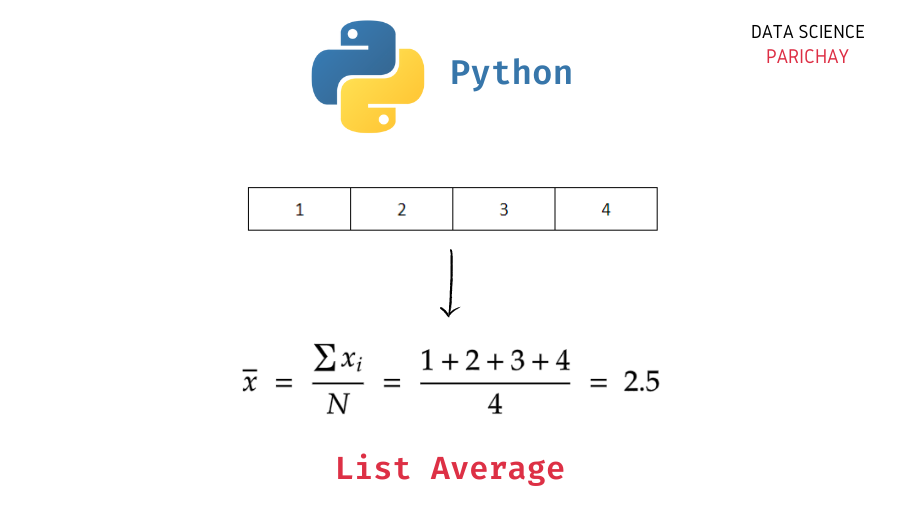# Python – Find Average of values in a List

Lists are a very versatile data structure in Python. When working with a list of numbers, it can be helpful to know how to calculate the mean of list values quickly. For example, you have a list of test scores and want to know what the average score was for the test. In this tutorial, we will look at how to get the average of a list in Python with the help of some examples.

You can use a combination of Python `sum()` and `len()` functions to compute the mean of a list. Alternatively, you can also use methods defined in libraries such as `statistics`, `numpy`, etc. to get the average of a list of values.

Let’s look at the above-mentioned methods with the help of examples.

To compute the mean of a list, you can use the `sum()` function to get the sum of the values in the list and divide that with the length of the list returned from the `len()` function.

```# create a list
ls = [1,2,3,4]
# average of list
sum(ls) / len(ls)```

Output:

`2.5`

We get 2.5 as the average for the list above list of values.

You can also use the `statistics` standard library in Python to get the mean of a list. Pass the list as an argument to the `statistics.mean()` function.

```import statistics

# create a list
ls = [1,2,3,4]
# average of list
statistics.mean(ls)```

Output:

📚 Data Science Programs By Skill Level

Introductory

Intermediate ⭐⭐⭐

🔎 Find Data Science Programs 👨‍💻 111,889 already enrolled

Disclaimer: Data Science Parichay is reader supported. When you purchase a course through a link on this site, we may earn a small commission at no additional cost to you. Earned commissions help support this website and its team of writers.

`2.5`

We get the same result as above.

For more on the `statistics` library, refer to its documentation.

You can also use the `numpy` library to get the list average. Numpy has a number of useful functions for working with arrays in Python.

```import numpy as np

# create a list
ls = [1,2,3,4]
# average of list
np.mean(ls)```

Output:

`2.5`

We get 2.5 as the average.

You might also be interested in –

•